# Selina Solutions Concise Maths Class 7 Chapter 11: Fundamental Concepts (Including Fundamental Operations) Exercise 11C

Selina Solutions Concise Maths Class 7 Chapter 11 Fundamental Concepts (Including Fundamental Operations) Exercise 11C has answers curated by highly experienced faculty at BYJU’S. This exercise deals with multiplication operations on monomial and polynomial, as per the latest syllabus of ICSE board. Students are advised to solve the exercise problems using the solutions PDF as a major reference guide. Selina Solutions Concise Maths Class 7 Chapter 11 Fundamental Concepts (Including Fundamental Operations) Exercise 11C, PDF links are available here with a free download option.

## Selina Solutions Concise Maths Class 7 Chapter 11: Fundamental Concepts (Including Fundamental Operations) Exercise 11C Download PDF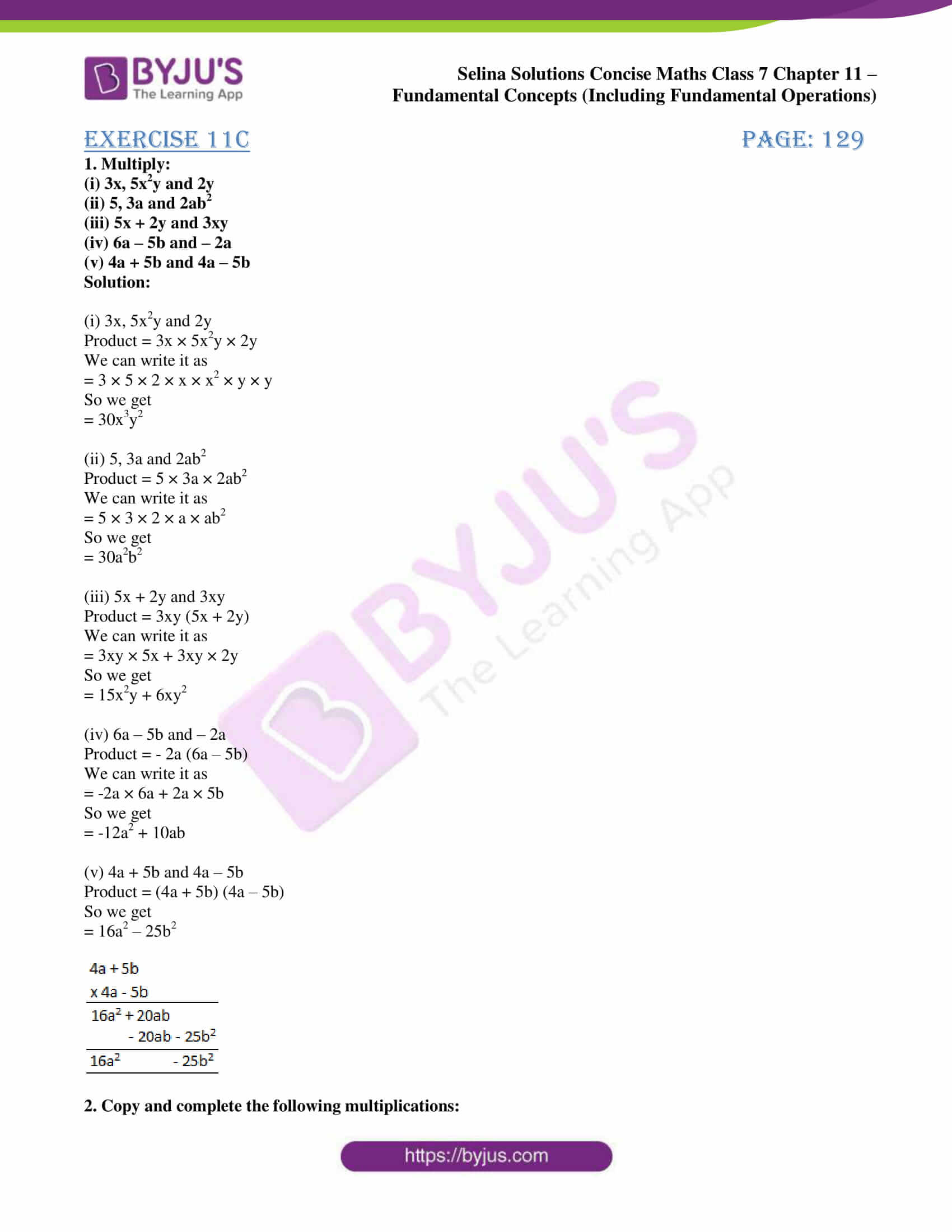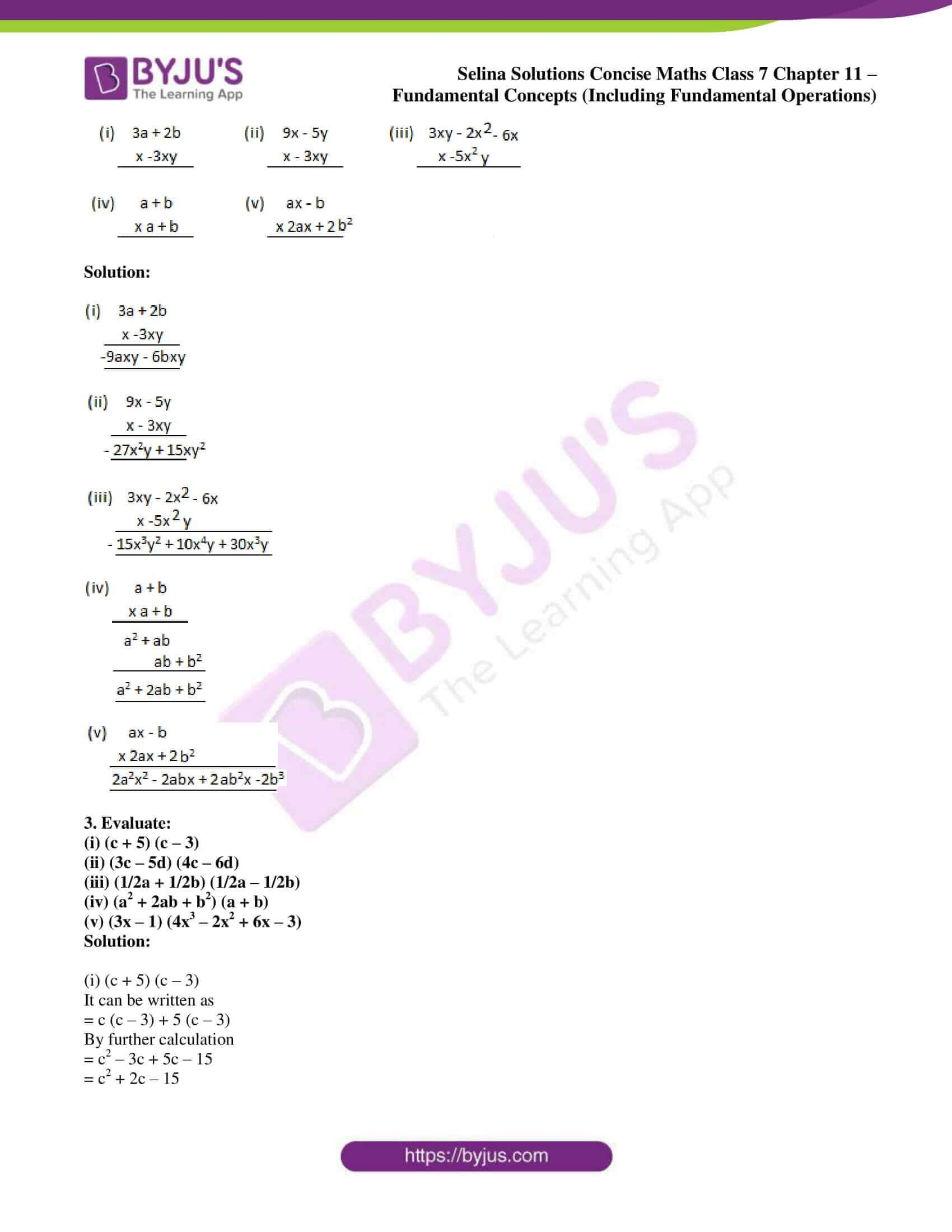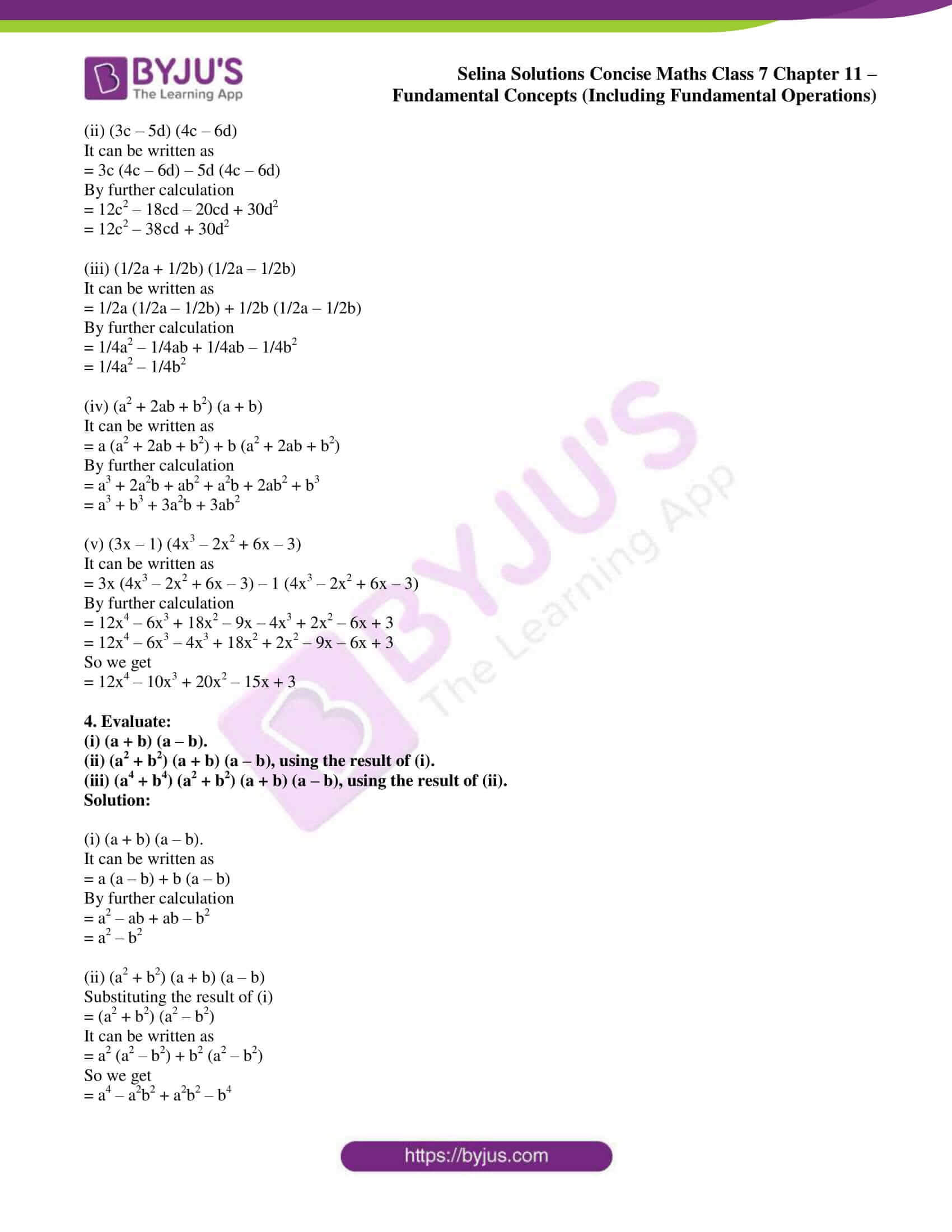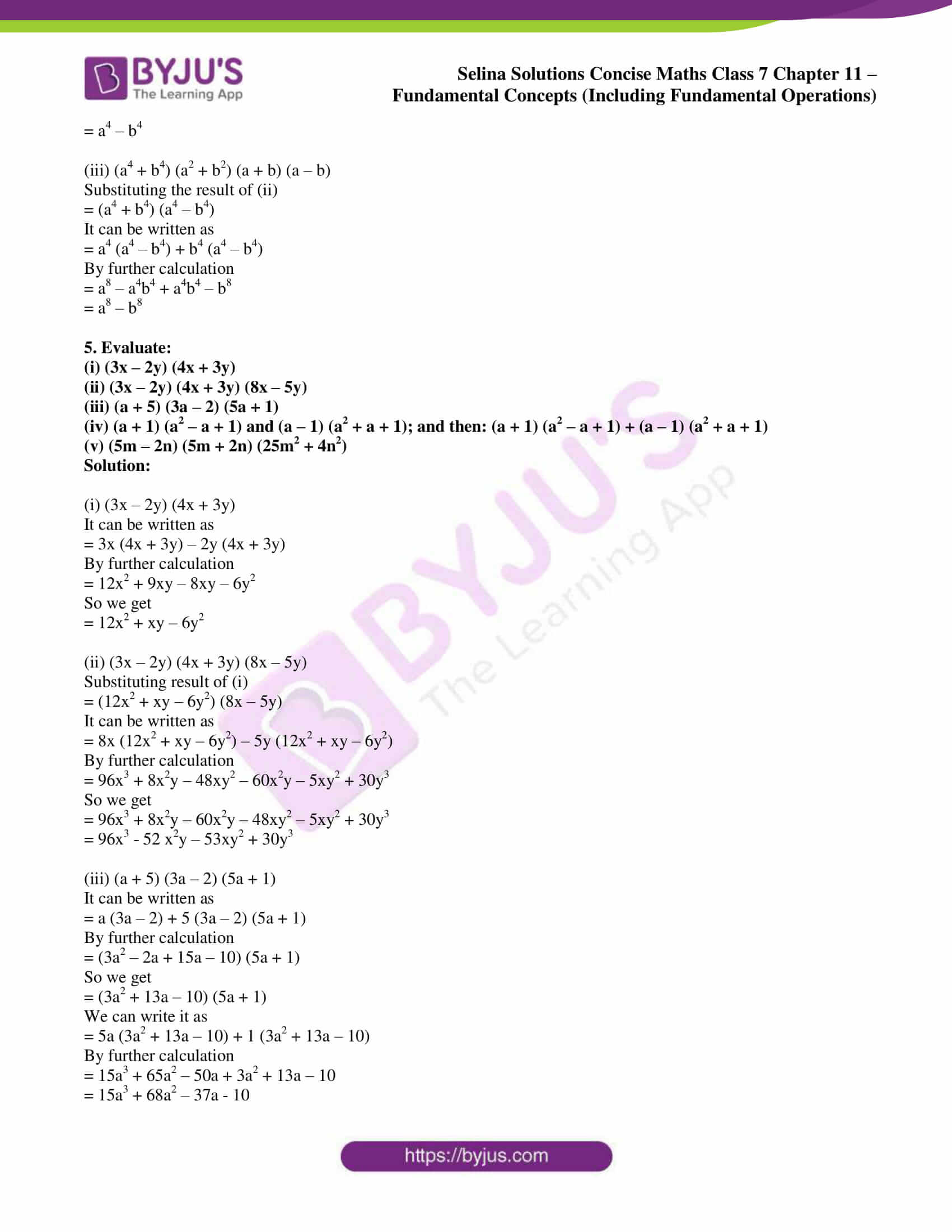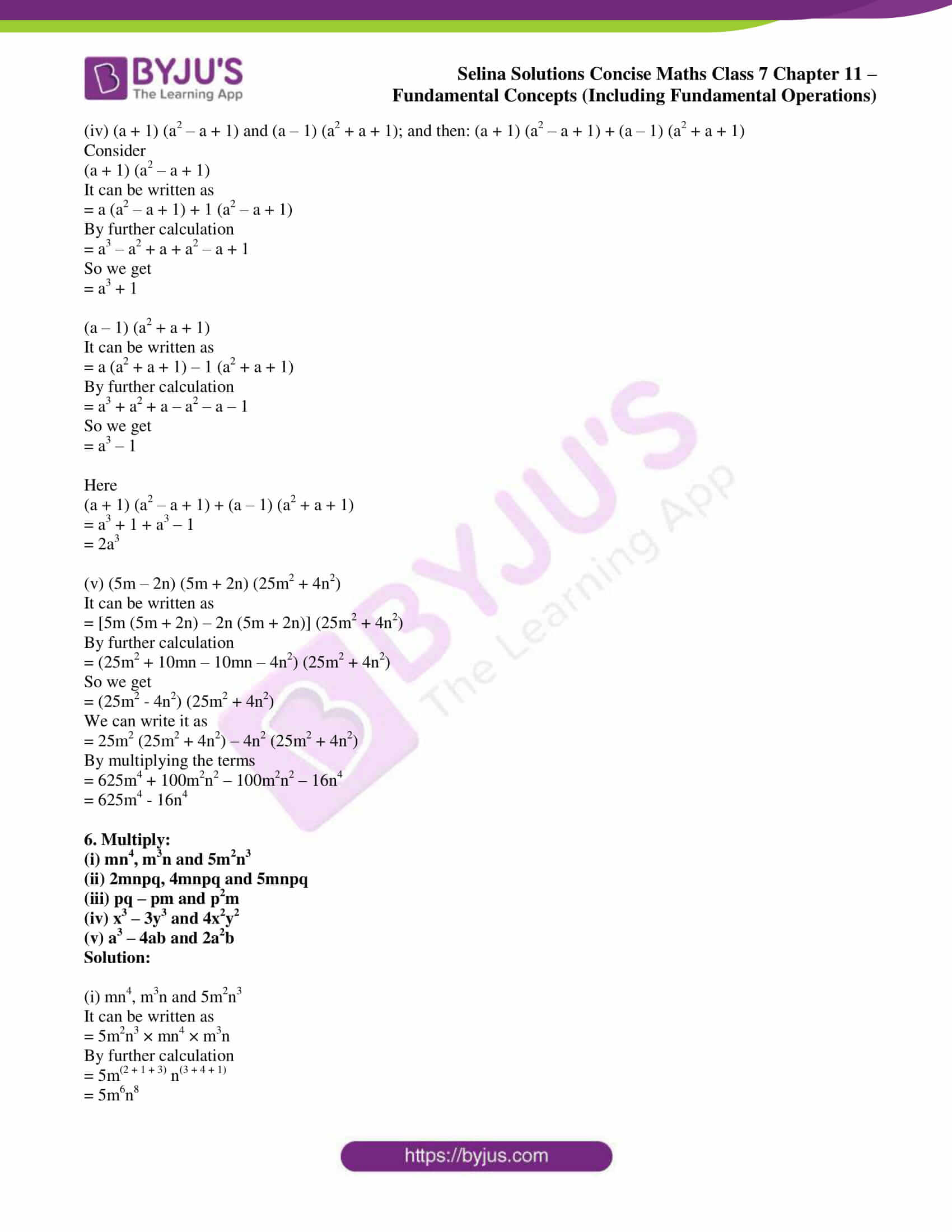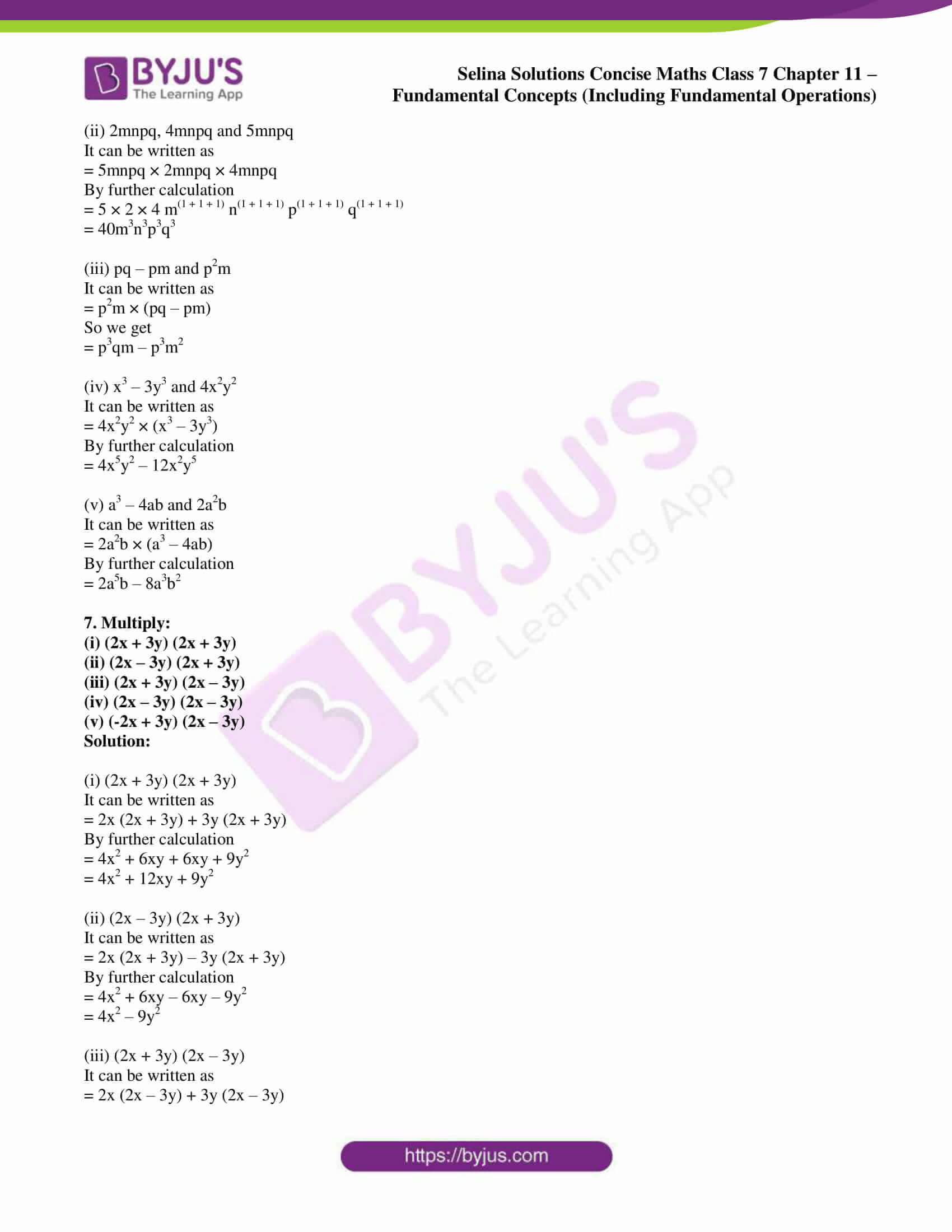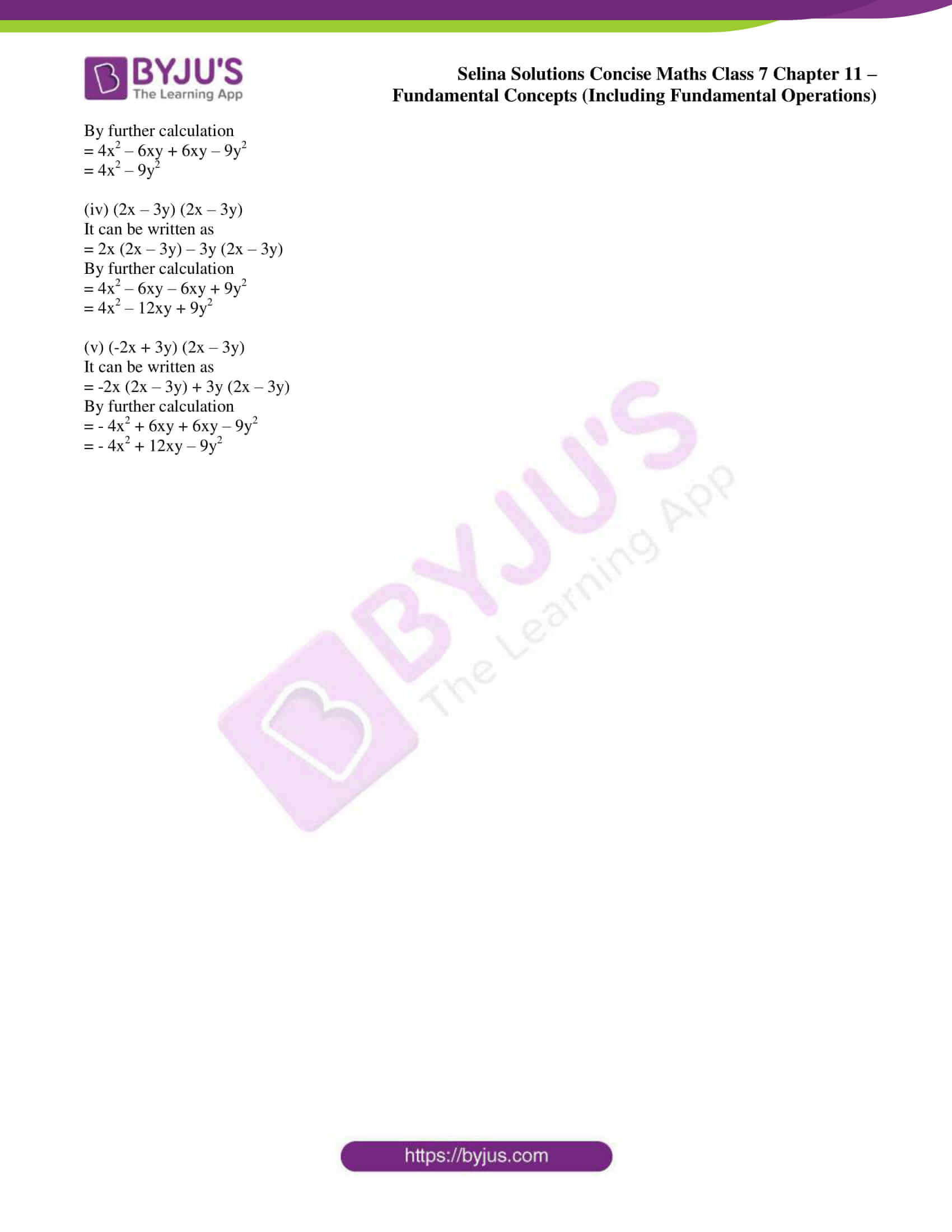### Access other exercises of Selina Solutions Concise Maths Class 7 Chapter 11: Fundamental Concepts (Including Fundamental Operations)

Exercise 11A Solutions

Exercise 11B Solutions

Exercise 11D Solutions

Exercise 11E Solutions

Exercise 11F Solutions

### Access Selina Solutions Concise Maths Class 7 Chapter 11: Fundamental Concepts (Including Fundamental Operations) Exercise 11C

#### Exercise 11C page: 129

1. Multiply:

(i) 3x, 5x2y and 2y

(ii) 5, 3a and 2ab2

(iii) 5x + 2y and 3xy

(iv) 6a – 5b and – 2a

(v) 4a + 5b and 4a – 5b

Solution:

(i) 3x, 5x2y and 2y

Product = 3x × 5x2y × 2y

We can write it as

= 3 × 5 × 2 × x × x2 × y × y

So we get

= 30x3y2

(ii) 5, 3a and 2ab2

Product = 5 × 3a × 2ab2

We can write it as

= 5 × 3 × 2 × a × ab2

So we get

= 30a2b2

(iii) 5x + 2y and 3xy

Product = 3xy (5x + 2y)

We can write it as

= 3xy × 5x + 3xy × 2y

So we get

= 15x2y + 6xy2

(iv) 6a – 5b and – 2a

Product = – 2a (6a – 5b)

We can write it as

= -2a × 6a + 2a × 5b

So we get

= -12a2 + 10ab

(v) 4a + 5b and 4a – 5b

Product = (4a + 5b) (4a – 5b)

So we get

= 16a2 – 25b2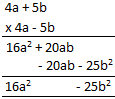2. Copy and complete the following multiplications:Solution: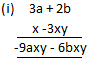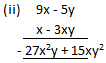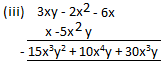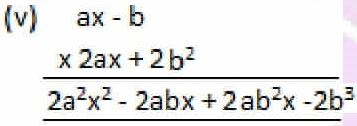3. Evaluate:

(i) (c + 5) (c – 3)

(ii) (3c – 5d) (4c – 6d)

(iii) (1/2a + 1/2b) (1/2a – 1/2b)

(iv) (a2 + 2ab + b2) (a + b)

(v) (3x – 1) (4x3 – 2x2 + 6x – 3)

Solution:

(i) (c + 5) (c – 3)

It can be written as

= c (c – 3) + 5 (c – 3)

By further calculation

= c2 – 3c + 5c – 15

= c2 + 2c – 15

(ii) (3c – 5d) (4c – 6d)

It can be written as

= 3c (4c – 6d) – 5d (4c – 6d)

By further calculation

= 12c2 – 18cd – 20cd + 30d2

= 12c2 – 38cd + 30d2

(iii) (1/2a + 1/2b) (1/2a – 1/2b)

It can be written as

= 1/2a (1/2a – 1/2b) + 1/2b (1/2a – 1/2b)

By further calculation

= 1/4a2 – 1/4ab + 1/4ab – 1/4b2

= 1/4a2 – 1/4b2

(iv) (a2 + 2ab + b2) (a + b)

It can be written as

= a (a2 + 2ab + b2) + b (a2 + 2ab + b2)

By further calculation

= a3 + 2a2b + ab2 + a2b + 2ab2 + b3

= a3 + b3 + 3a2b + 3ab2

(v) (3x – 1) (4x3 – 2x2 + 6x – 3)

It can be written as

= 3x (4x3 – 2x2 + 6x – 3) – 1 (4x3 – 2x2 + 6x – 3)

By further calculation

= 12x4 – 6x3 + 18x2 – 9x – 4x3 + 2x2 – 6x + 3

= 12x4 – 6x3 – 4x3 + 18x2 + 2x2 – 9x – 6x + 3

So we get

= 12x4 – 10x3 + 20x2 – 15x + 3

4. Evaluate:

(i) (a + b) (a – b).

(ii) (a2 + b2) (a + b) (a – b), using the result of (i).

(iii) (a4 + b4) (a2 + b2) (a + b) (a – b), using the result of (ii).

Solution:

(i) (a + b) (a – b).

It can be written as

= a (a – b) + b (a – b)

By further calculation

= a2 – ab + ab – b2

= a2 – b2

(ii) (a2 + b2) (a + b) (a – b)

Substituting the result of (i)

= (a2 + b2) (a2 – b2)

It can be written as

= a2 (a2 – b2) + b2 (a2 – b2)

So we get

= a4 – a2b2 + a2b2 – b4

= a4 – b4

(iii) (a4 + b4) (a2 + b2) (a + b) (a – b)

Substituting the result of (ii)

= (a4 + b4) (a4 – b4)

It can be written as

= a4 (a4 – b4) + b4 (a4 – b4)

By further calculation

= a8 – a4b4 + a4b4 – b8

= a8 – b8

5. Evaluate:

(i) (3x – 2y) (4x + 3y)

(ii) (3x – 2y) (4x + 3y) (8x – 5y)

(iii) (a + 5) (3a – 2) (5a + 1)

(iv) (a + 1) (a2 – a + 1) and (a – 1) (a2 + a + 1); and then: (a + 1) (a2 – a + 1) + (a – 1) (a2 + a + 1)

(v) (5m – 2n) (5m + 2n) (25m2 + 4n2)

Solution:

(i) (3x – 2y) (4x + 3y)

It can be written as

= 3x (4x + 3y) – 2y (4x + 3y)

By further calculation

= 12x2 + 9xy – 8xy – 6y2

So we get

= 12x2 + xy – 6y2

(ii) (3x – 2y) (4x + 3y) (8x – 5y)

Substituting result of (i)

= (12x2 + xy – 6y2) (8x – 5y)

It can be written as

= 8x (12x2 + xy – 6y2) – 5y (12x2 + xy – 6y2)

By further calculation

= 96x3 + 8x2y – 48xy2 – 60x2y – 5xy2 + 30y3

So we get

= 96x3 + 8x2y – 60x2y – 48xy2 – 5xy2 + 30y3

= 96x3 – 52 x2y – 53xy2 + 30y3

(iii) (a + 5) (3a – 2) (5a + 1)

It can be written as

= a (3a – 2) + 5 (3a – 2) (5a + 1)

By further calculation

= (3a2 – 2a + 15a – 10) (5a + 1)

So we get

= (3a2 + 13a – 10) (5a + 1)

We can write it as

= 5a (3a2 + 13a – 10) + 1 (3a2 + 13a – 10)

By further calculation

= 15a3 + 65a2 – 50a + 3a2 + 13a – 10

= 15a3 + 68a2 – 37a – 10

(iv) (a + 1) (a2 – a + 1) and (a – 1) (a2 + a + 1); and then: (a + 1) (a2 – a + 1) + (a – 1) (a2 + a + 1)

Consider

(a + 1) (a2 – a + 1)

It can be written as

= a (a2 – a + 1) + 1 (a2 – a + 1)

By further calculation

= a3 – a2 + a + a2 – a + 1

So we get

= a3 + 1

(a – 1) (a2 + a + 1)

It can be written as

= a (a2 + a + 1) – 1 (a2 + a + 1)

By further calculation

= a3 + a2 + a – a2 – a – 1

So we get

= a3 – 1

Here

(a + 1) (a2 – a + 1) + (a – 1) (a2 + a + 1)

= a3 + 1 + a3 – 1

= 2a3

(v) (5m – 2n) (5m + 2n) (25m2 + 4n2)

It can be written as

= [5m (5m + 2n) – 2n (5m + 2n)] (25m2 + 4n2)

By further calculation

= (25m2 + 10mn – 10mn – 4n2) (25m2 + 4n2)

So we get

= (25m2 – 4n2) (25m2 + 4n2)

We can write it as

= 25m2 (25m2 + 4n2) – 4n2 (25m2 + 4n2)

By multiplying the terms

= 625m4 + 100m2n2 – 100m2n2 – 16n4

= 625m4 – 16n4

6. Multiply:

(i) mn4, m3n and 5m2n3

(ii) 2mnpq, 4mnpq and 5mnpq

(iii) pq – pm and p2m

(iv) x3 – 3y3 and 4x2y2

(v) a3 – 4ab and 2a2b

Solution:

(i) mn4, m3n and 5m2n3

It can be written as

= 5m2n3 × mn4 × m3n

By further calculation

= 5m(2 + 1 + 3) n(3 + 4 + 1)

= 5m6n8

(ii) 2mnpq, 4mnpq and 5mnpq

It can be written as

= 5mnpq × 2mnpq × 4mnpq

By further calculation

= 5 × 2 × 4 m(1 + 1 + 1) n(1 + 1 + 1) p(1 + 1 + 1) q(1 + 1 + 1)

= 40m3n3p3q3

(iii) pq – pm and p2m

It can be written as

= p2m × (pq – pm)

So we get

= p3qm – p3m2

(iv) x3 – 3y3 and 4x2y2

It can be written as

= 4x2y2 × (x3 – 3y3)

By further calculation

= 4x5y2 – 12x2y5

(v) a3 – 4ab and 2a2b

It can be written as

= 2a2b × (a3 – 4ab)

By further calculation

= 2a5b – 8a3b2

7. Multiply:

(i) (2x + 3y) (2x + 3y)

(ii) (2x – 3y) (2x + 3y)

(iii) (2x + 3y) (2x – 3y)

(iv) (2x – 3y) (2x – 3y)

(v) (-2x + 3y) (2x – 3y)

Solution:

(i) (2x + 3y) (2x + 3y)

It can be written as

= 2x (2x + 3y) + 3y (2x + 3y)

By further calculation

= 4x2 + 6xy + 6xy + 9y2

= 4x2 + 12xy + 9y2

(ii) (2x – 3y) (2x + 3y)

It can be written as

= 2x (2x + 3y) – 3y (2x + 3y)

By further calculation

= 4x2 + 6xy – 6xy – 9y2

= 4x2 – 9y2

(iii) (2x + 3y) (2x – 3y)

It can be written as

= 2x (2x – 3y) + 3y (2x – 3y)

By further calculation

= 4x2 – 6xy + 6xy – 9y2

= 4x2 – 9y2

(iv) (2x – 3y) (2x – 3y)

It can be written as

= 2x (2x – 3y) – 3y (2x – 3y)

By further calculation

= 4x2 – 6xy – 6xy + 9y2

= 4x2 – 12xy + 9y2

(v) (-2x + 3y) (2x – 3y)

It can be written as

= -2x (2x – 3y) + 3y (2x – 3y)

By further calculation

= – 4x2 + 6xy + 6xy – 9y2

= – 4x2 + 12xy – 9y2